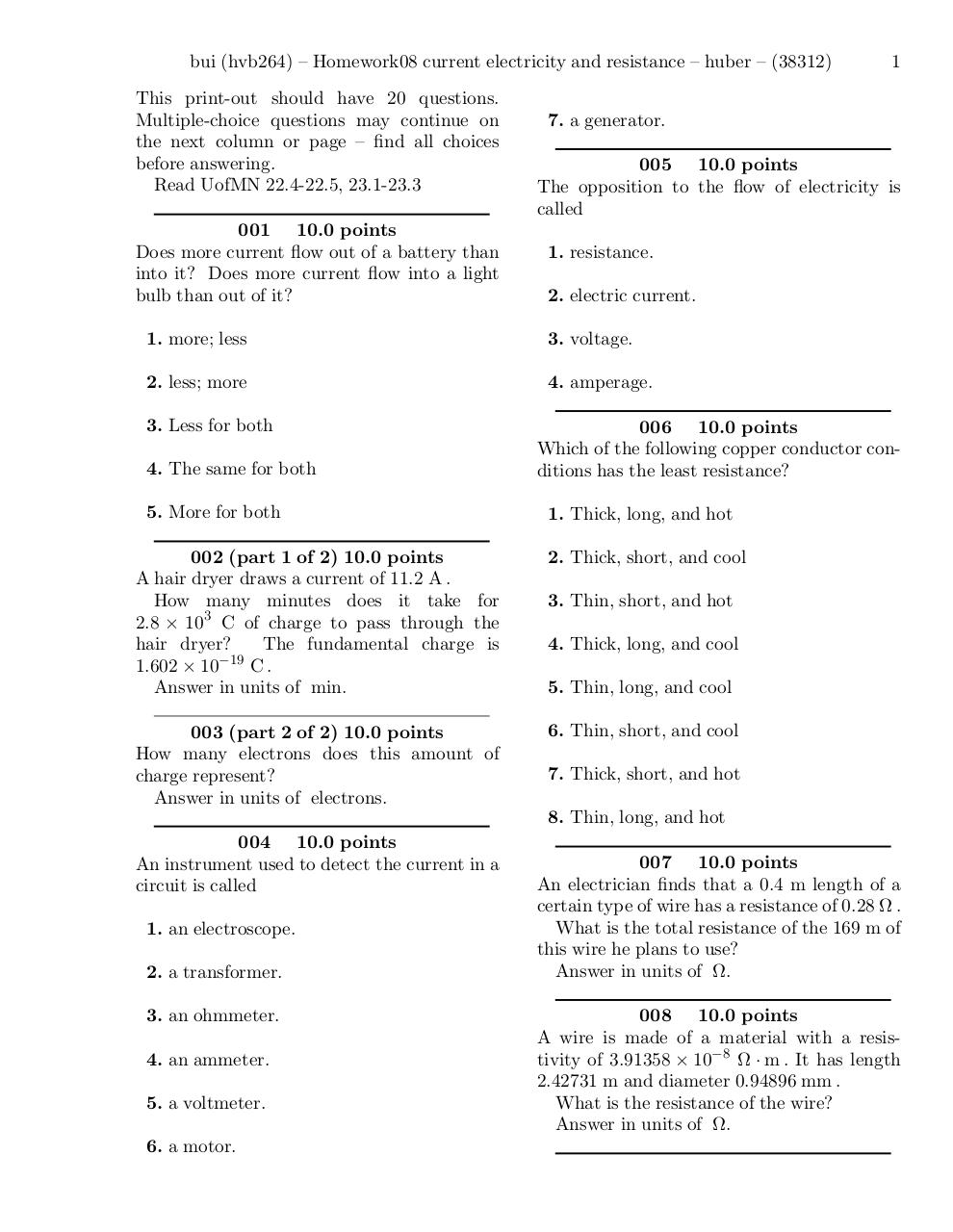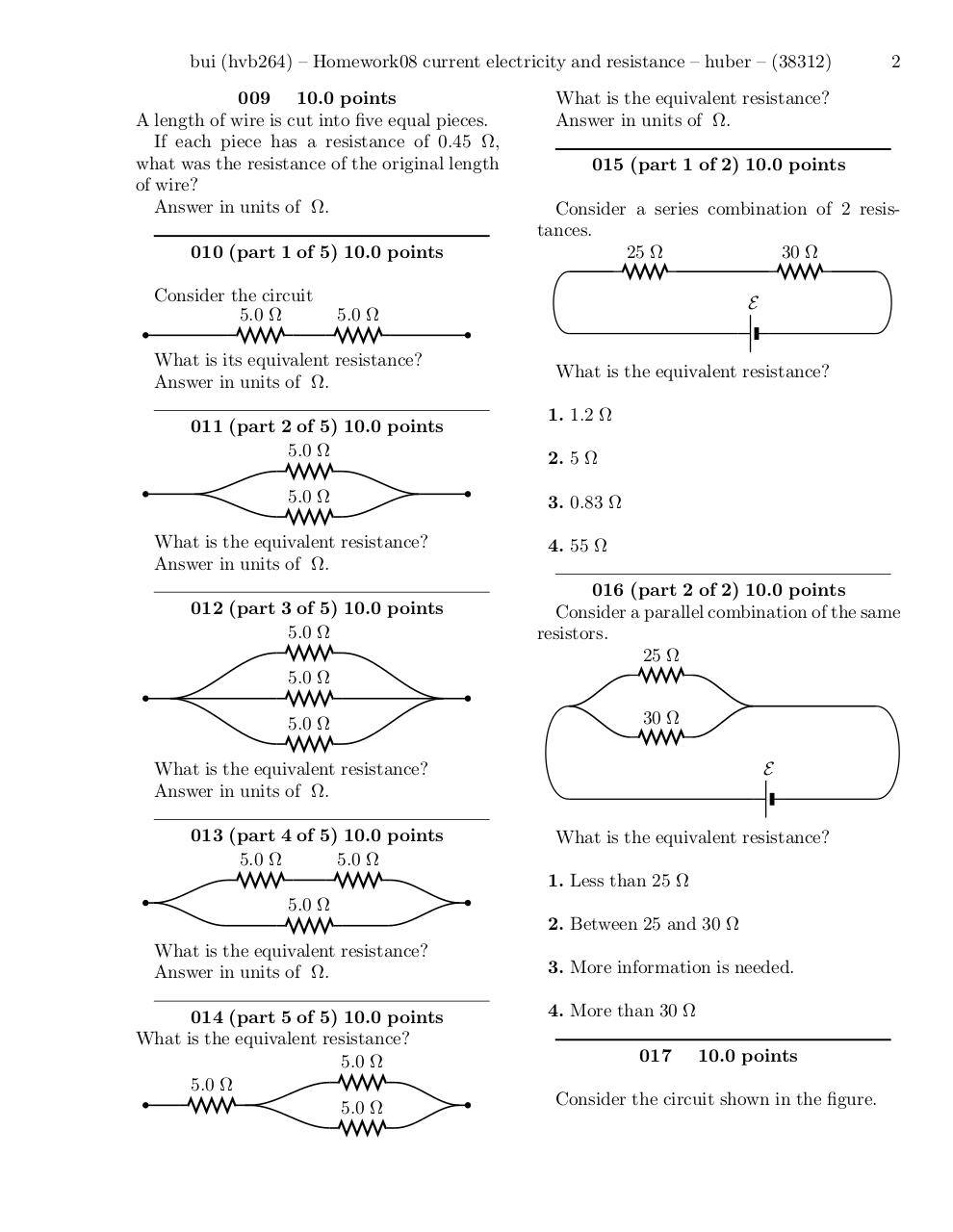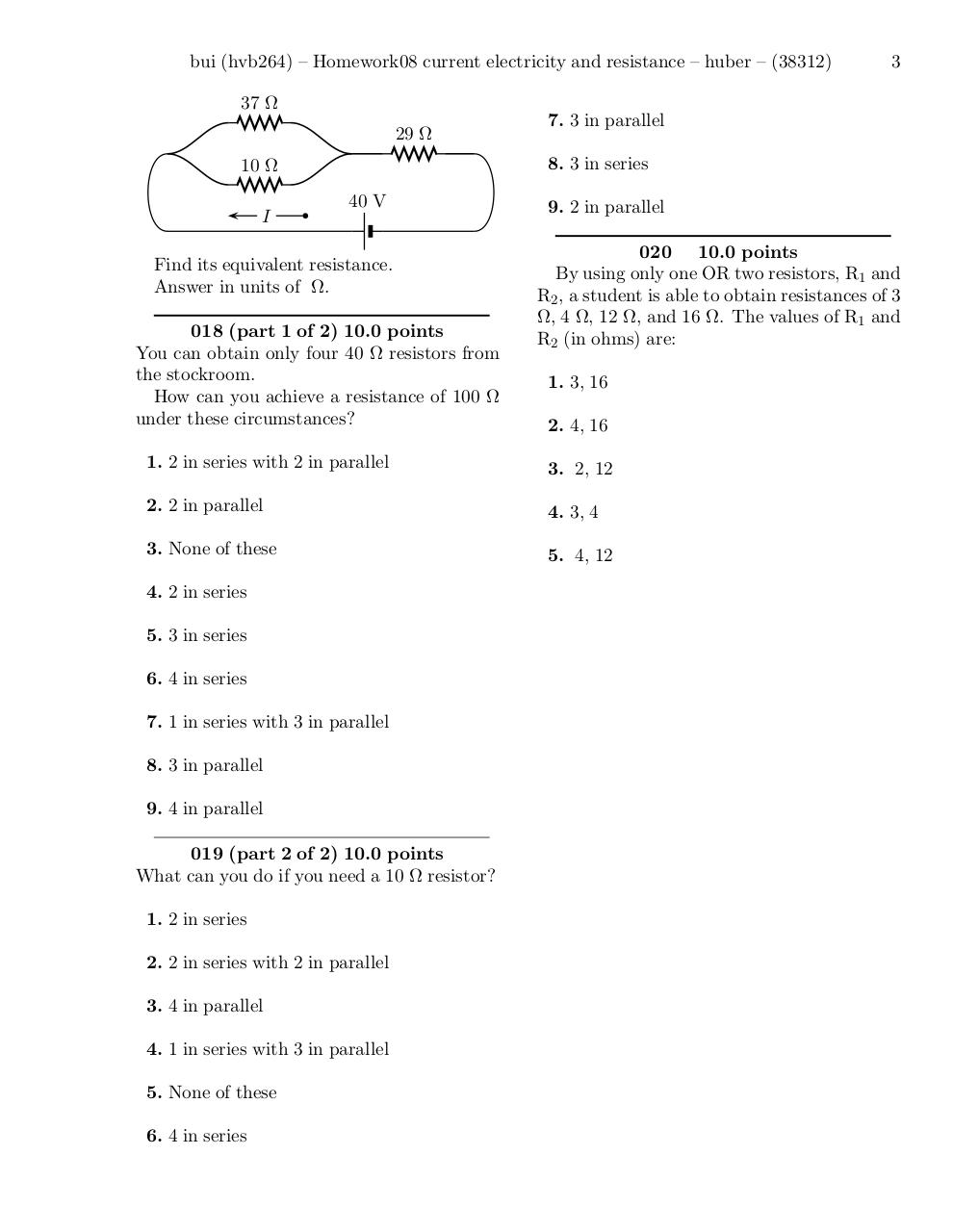# Homework08 current electricity and resistance problems .pdf

### File information

Original filename: Homework08 current electricity and resistance-problems.pdf
Title: /tmp/576-14888325827693443-29196-36273.dvi

This PDF 1.4 document has been generated by dvips(k) 5.98 Copyright 2009 Radical Eye Software / GPL Ghostscript 8.70, and has been sent on pdf-archive.com on 08/03/2017 at 01:40, from IP address 66.41.x.x. The current document download page has been viewed 262 times.
File size: 31 KB (3 pages).
Privacy: public file

Homework08 current electricity and resistance-problems.pdf (PDF, 31 KB)

### Document preview

bui (hvb264) – Homework08 current electricity and resistance – huber – (38312)
This print-out should have 20 questions.
Multiple-choice questions may continue on
the next column or page – find all choices
001 10.0 points
Does more current flow out of a battery than
into it? Does more current flow into a light
bulb than out of it?

7. a generator.
005 10.0 points
The opposition to the flow of electricity is
called
1. resistance.
2. electric current.

1. more; less

3. voltage.

2. less; more

4. amperage.

3. Less for both
4. The same for both
5. More for both

1

006 10.0 points
Which of the following copper conductor conditions has the least resistance?
1. Thick, long, and hot

002 (part 1 of 2) 10.0 points
A hair dryer draws a current of 11.2 A .
How many minutes does it take for
2.8 × 103 C of charge to pass through the
hair dryer?
The fundamental charge is
1.602 × 10−19 C .

2. Thick, short, and cool

003 (part 2 of 2) 10.0 points
How many electrons does this amount of
charge represent?

6. Thin, short, and cool

3. Thin, short, and hot
4. Thick, long, and cool
5. Thin, long, and cool

7. Thick, short, and hot
8. Thin, long, and hot

004 10.0 points
An instrument used to detect the current in a
circuit is called
1. an electroscope.
2. a transformer.
3. an ohmmeter.
4. an ammeter.
5. a voltmeter.
6. a motor.

007 10.0 points
An electrician finds that a 0.4 m length of a
certain type of wire has a resistance of 0.28 Ω .
What is the total resistance of the 169 m of
this wire he plans to use?
008 10.0 points
A wire is made of a material with a resistivity of 3.91358 × 10−8 Ω · m . It has length
2.42731 m and diameter 0.94896 mm .
What is the resistance of the wire?

bui (hvb264) – Homework08 current electricity and resistance – huber – (38312)
009 10.0 points
A length of wire is cut into five equal pieces.
If each piece has a resistance of 0.45 Ω,
what was the resistance of the original length
of wire?
010 (part 1 of 5) 10.0 points
Consider the circuit
5.0 Ω

What is the equivalent resistance?
015 (part 1 of 2) 10.0 points
Consider a series combination of 2 resistances.
25 Ω
30 Ω
E

5.0 Ω

What is its equivalent resistance?
011 (part 2 of 5) 10.0 points
5.0 Ω
5.0 Ω
What is the equivalent resistance?
012 (part 3 of 5) 10.0 points
5.0 Ω

What is the equivalent resistance?
1. 1.2 Ω
2. 5 Ω
3. 0.83 Ω
4. 55 Ω
016 (part 2 of 2) 10.0 points
Consider a parallel combination of the same
resistors.
25 Ω

5.0 Ω
5.0 Ω

30 Ω

What is the equivalent resistance?
013 (part 4 of 5) 10.0 points
5.0 Ω
5.0 Ω

E

What is the equivalent resistance?
1. Less than 25 Ω

5.0 Ω
2. Between 25 and 30 Ω
What is the equivalent resistance?
014 (part 5 of 5) 10.0 points
What is the equivalent resistance?
5.0 Ω
5.0 Ω
5.0 Ω

2

4. More than 30 Ω
017

10.0 points

Consider the circuit shown in the figure.

bui (hvb264) – Homework08 current electricity and resistance – huber – (38312)
37 Ω
29 Ω

7. 3 in parallel
8. 3 in series

10 Ω
I

40 V

Find its equivalent resistance.
018 (part 1 of 2) 10.0 points
You can obtain only four 40 Ω resistors from
the stockroom.
How can you achieve a resistance of 100 Ω
under these circumstances?

9. 2 in parallel
020 10.0 points
By using only one OR two resistors, R1 and
R2 , a student is able to obtain resistances of 3
Ω, 4 Ω, 12 Ω, and 16 Ω. The values of R1 and
R2 (in ohms) are:
1. 3, 16
2. 4, 16

1. 2 in series with 2 in parallel

3. 2, 12

2. 2 in parallel

4. 3, 4

3. None of these

5. 4, 12

4. 2 in series
5. 3 in series
6. 4 in series
7. 1 in series with 3 in parallel
8. 3 in parallel
9. 4 in parallel
019 (part 2 of 2) 10.0 points
What can you do if you need a 10 Ω resistor?
1. 2 in series
2. 2 in series with 2 in parallel
3. 4 in parallel
4. 1 in series with 3 in parallel
5. None of these
6. 4 in series

3#### HTML Code

Copy the following HTML code to share your document on a Website or Blog

#### QR Code### Related keywords